Experiment 3: We now have the same set up except that the railroad car is now moving to the left with a velocity v.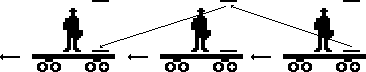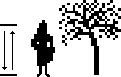Question: What happens?

Answer: From Mr. Einstein's perspective, the beam of light keeps going up and down between the mirrors, but from Mrs. Einstein's perspective, the light now has to travel a diagonal path from one mirror to the other. Since Mrs. Einstein still measures the speed of light as c, she is now going to observe Mr. Einstein's light clock as ticking slower than hers since the light now has a longer distance to travel. However, since Mr. Einstein still experiences his watch as being synchronized with his clock, Mrs. Einstein will see his watch slow down along with his clock!

Conclusion: If someone moves in a straight line with velocity v with respect to you, then you will observe time passing more slowly for them.

With a little algebra we can compute exactly how much time will slow down. Let,

L= length or height of the light clocks
v = Mr. Einstein's velocity
t = time between ticks on Mrs. Einstein's clock
t´ = time between ticks on Mr. Einstein's clock as Mrs. Einstein observes it
c = speed of light.

We will make frequent use of the formula distance = rate x time. We now compute the distance that the light travels in two ways.On the one hand, the distance traveled is ct´ (distance = rate x time). But on the other hand, using the Pythagorean Theorem, we have that the distance is

2= 2= 2==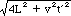.

Hence, ct´ =which implies that c22 = 4L2 + v22 ,

which implies c22 - v22 = 4L2 ,

which implies t´2(c2 - v2) = 4L2,

which implies t´2 =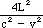=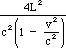which implies t´ ==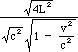=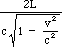.

Since t = (2L)/c (i.e. t = d/r), this gives us

t´ =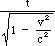Therefore, if t is the time between ticks on Mrs. Einstein's watch, then she will observe a longer interval of

t´ =between ticks on Mr. Einstein's watch! Notice that this difference is not very much unless one is traveling at an extremely fast velocity.